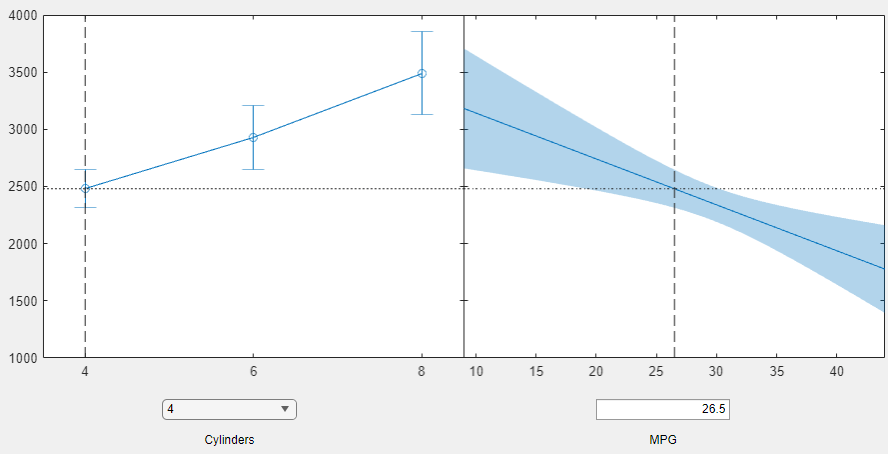# plotSlice

Plot of slices through fitted generalized linear regression surface

## Syntax

```plotSlice(mdl) h = plotSlice(mdl) ```

## Description

`plotSlice(mdl)` creates a new figure containing a series of plots, each representing a slice through the regression surface predicted by `mdl`. For each plot, the surface slice is shown as a function of a single predictor variable, with the other predictor variables held constant.

`h = plotSlice(mdl)` returns handles to the lines in the plot.

## Input Arguments

 `mdl` Generalized linear model, specified as a full `GeneralizedLinearModel` object constructed using `fitglm` or `stepwiseglm`, or a compacted `CompactGeneralizedLinearModel` object constructed using `compact`.

## Output Arguments

 `h` Vector of handles to lines or patches in the plot.

## Examples

expand all

Create a slice plot of a Poisson generalized linear model.

Generate artificial data for the model using Poisson random numbers with two underlying predictors `X(1)` and `X(2)`.

```rng('default') % for reproducibility rndvars = randn(100,2); X = [2+rndvars(:,1),rndvars(:,2)]; mu = exp(1 + X*[1;2]); y = poissrnd(mu);```

Create a generalized linear regression model of Poisson data.

`mdl = fitglm(X,y,'y ~ x1 + x2','Distribution','poisson');`

Create the slice plot.

`plotSlice(mdl)`Drag the `x1` prediction line to the right and view the changes in the prediction and the response curve for the `x2` predictor.## Tips

• If there are more than eight predictors, `plotSlice` selects the first five for plotting. Use the Predictors menu to control which predictors are plotted.

• The Bounds menu lets you choose between simultaneous or non-simultaneous bounds, and between bounds on the function or bounds on a new observation.